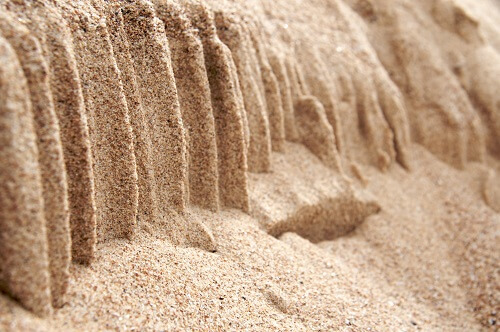# Sand Unit Weight or Specific Weight - Calculation

The first thing is Unit weight is as same as Specific Weight. And the specific weight of a material is actually the product of its density and the standard gravity.

The density of the material is the per unit volume, calculated in the unit of kg/mor lb/ft(pcf). The standard gravity is usually given in m/sof ft/s2, and on Earth usually taken as 9.81 m/s2.

In this article, details about unit weight of sand, how to determine it, and typical unit weight values of different sand types will be presented.

## Specific Weight or Unit weight of Sand

Unit weight or Specific Weight of sand is calculated by the product of the density of sand and the standard gravity of sand.

According to the US customary measurement system, dry weighs 1.631 gram per cubic centimeter, this density is equal to 101.8 pounds per cubic foot [lb/ft³].### Different Sand Type Density

The following table represents the typical dry density values for different types of sand.

 Sand Type Dry Density (g/cm3) Sand 1.52 Sandy Loam 1.44 Silt Loam 1.28 Clay Loam 1.28 Loam 1.36 Clay 1.20 Concrete 2.40

In another word, the Unit weight of sand is the ratio of the total weight of sand to the total volume of sand.

Unit Weight, is usually determined in the laboratory by measuring the weight and volume of a relatively undisturbed soil sample obtained from a brass ring. Measuring the unit weight of soil in the field may consist of a sand cone test, rubber balloon, or nuclear densometer.

### How to Calculate the Unit Weight of Sand

To calculate the unit weight of sand, we have to know some information, the volume of one unit of sand, the composition of the sand, and the mass densities of each component. Sand weight calculator also has to be taken.

• First: We have to know the constituent materials of the substance. In the Sand, assumed it was a mixture of the minerals olivine and basalt. For other types of sand, may have mixtures of quartz, gypsum, or silica.
• Second: We have to know the volumetric percentage of each constituent material in one unit of the substance.
• Third: We have to know the mass densities (or specific gravity) of the constituent materials.
• Fourth: From the volume and the mass densities, now we can determine the mass of each material. Now by adding the masses to get the total mass of the substance, which is proportional to the total weight.

### Unit Weight of Different Types of Sand

The followings are the typical value of different types of sand.

 Sand Type Unit Weight (Kg/m3) Sand (dry) 1540-1600 Sand (wet) 1760-2000 Chalk 2100 Clay Sand 1900 Clinker 750 Ashes 650 Bricks Broken 1420

### Disclaimer

Please note that the information in Civiltoday.com is designed to provide general information on the topics presented. The information provided should not be used as a substitute for professional services.

### Subscribe Us

To get regular update and new article notification please subscribe us.